Next: An example of the Up: SPECTRAL FLUCTUATIONS Previous: SPECTRAL FLUCTUATIONS

## Paradox: large n vs. the ensemble average

Now for the paradox. Imaginein Figure 8. Will we see the same limit as results from the ensemble average? Here are two contradictory points of view:

• For increasing n, the fluctuations on the nonzero autocorrelation lags get smaller, so the autocorrelation should tend to an impulse function. Its Fourier transform, the spectrum, should tend to a constant.
• On the other hand, for increasing n, as in Figure 3, the spectrum does not get any smoother, because the FTs should still look like random noise.
We will see that the first idea contains a false assumption. The autocorrelation does tend to an impulse, but the fuzz around the sides cannot be ignored--although the fuzz tends to zero amplitude, it also tends to infinite extent, and the product of zero with infinity here tends to have the same energy as the central impulse.

To examine this issue further, let us discover how these autocorrelations decrease to zero with n (the number of samples). Figure 9 shows the autocorrelation samples as a function of n in steps of n increasing by factors of four. Thus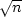increases by factors of two.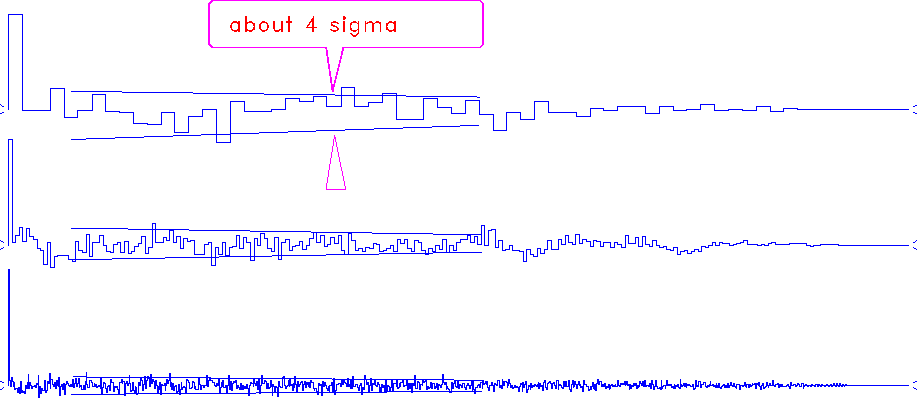fluct
Figure 9
Autocorrelation as a function of number of data points. The random-noise-series (even) lengths are 60, 240, 960.

Each autocorrelation in the figure was normalized at zero lag. We see the sample variance for nonzero lags of the autocorrelation dropping off as.We also observe that the ratios between the values for the first nonzero lags and the value at lag zero roughly fit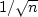.Notice also that the fluctuations drop off with lag. The drop-off goes to zero at a lag equal to the sample length, because the number of terms in the autocorrelation diminishes to zero at that lag. A first impression is that the autocorrelation fits a triangular envelope. More careful inspection, however, shows that the triangle bulges upward at wide offsets, or large values of k (this is slightly clearer in Figure 8).

Let us explain all these observations. Each lag of the autocorrelation is defined as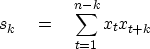(36)
where (xt) is a sequence of zero-mean independent random variables. Thus, the expectations of the autocorrelations can be easily computed:(37) (38)
In Figure 9, the value at lag zero is more or less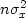(before normalization), the deviation being more or less the standard deviation (square root of the variance) of s0. On the other hand, for,as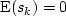, the value of the autocorrelation is directly the deviation of sk, i.e.,  something close to its standard deviation.

We now have to compute the variances of the sk. Let us write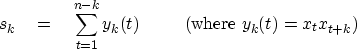(39)
So: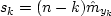, where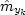is the sample mean of yk with n-k terms. If,, and we apply (33) to: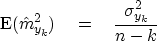(40)
The computation of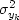is straightforward: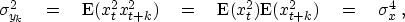(41)
Thus, for the autocorrelation sk: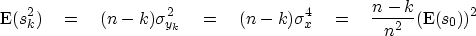(42)
Finally, as, we get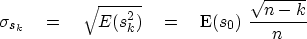(43)
This result explains the properties observed in Figure 9. As,all the nonzero lags tend to zero compared to the zero lag, since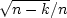tends to zero. Then, the first lags (k<<n) yield the ratiobetween the autocorrelations and the value at lag zero. Finally, the autocorrelations do not decrease linearly with k, because of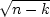.

We can now explain the paradox. The energy of the nonzero lags will be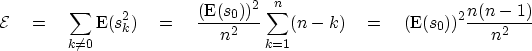(44)
Hence there is a conflict between the decrease to zero of the autocorrelations and the increasing number of nonzero lags, which themselves prevent the energy from decreasing to zero. The autocorrelation does not globally tend to an impulse function.

In the frequency domain, the spectrum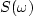is now(45)
So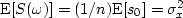,and the average spectrum is a constant, independent of the frequency. However, as the sk fluctuate more or less like,and as their count inis increasing with n, we will observe thatwill also fluctuate, and indeed,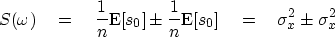(46)
This explains why the spectrum remains fuzzy: the fluctuation is independent of the number of samples, whereas the autocorrelation seems to tend to an impulse. In conclusion, the expectation (ensemble average) of the spectrum is not properly estimated by lettingin a sample.Next: An example of the Up: SPECTRAL FLUCTUATIONS Previous: SPECTRAL FLUCTUATIONS
Stanford Exploration Project
10/21/1998